# Action

(diff) ← Older revision | Latest revision (diff) | Newer revision → (diff)

A functional expressed by the definite integral of a function, the stationary values of which determine a real motion of a mechanical system acted upon by given active forces, in the class of kinematically possible motions between some two final positions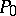andin space and satisfying certain conditions.

One distinguishes between Hamiltonian, Lagrangian and Jacobian actions, which appear in the corresponding principles of stationary action.

The Hamiltonian actionis defined in the class of kinematically possible motions of a holonomic system for which the initial and the final positions of the system, as well as the time of motion between them, are the same as the respective ones for real motion.

The Lagrangian action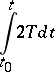and the Jacobian action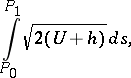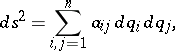are defined in the class of kinematically possible motions of a holonomic conservative system for which the initial and the final positions of the system, and the constant energy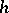, are the same as the respective magnitudes for a real motion. Hereis the kinetic energy of the system, and for a conservative system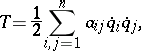whereare the generalized Lagrange coordinates, and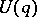is the force function of active forces.

How to Cite This Entry:
Action. Encyclopedia of Mathematics. URL: http://www.encyclopediaofmath.org/index.php?title=Action&oldid=13315
This article was adapted from an original article by V.V. Rumyantsev (originator), which appeared in Encyclopedia of Mathematics - ISBN 1402006098. See original article